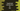# Introduction to Set in TypeScript with examples### Introduction to Set in TypeScript:

sets in typescript are similar to set in any other programming language. It is used to store distinct data, i.e. each value in a set can occur only once.

Set class of TypeScript provides a couple of different methods as well. In this post, we will learn how to create a set and its different methods with example.

### How to create a set:

We can create a set as like below:

``let firstSet = new Set();``

It creates a Set firstSet without any item. Set class provides a couple of methods that can be used to modify its items.

### Method and properties of Set class:

Following are the important methods and properties of Set class:

``````set.add() - add an item to the set
set.has() - check if an item is in the set
set.delete() - delete an item from the set
set.size - it returns the size of the set
set.clear() - it clears the set or it removes all items from the set``````

### Example to add items and delete items of a set:

Let’s take a look at the below example:

``````let firstSet = new Set();

console.log(firstSet.has('one'));
console.log(firstSet.size);

firstSet.delete('one');
console.log(firstSet.size);

firstSet.clear();

console.log(firstSet.size);``````

Here, we are using all the methods and properties shown above. It prints the below output:

``````true
3
2
0``````

### How to iterate through a set:

We can iterate through a set using a forEach or for..0f :

``````let firstSet = new Set();